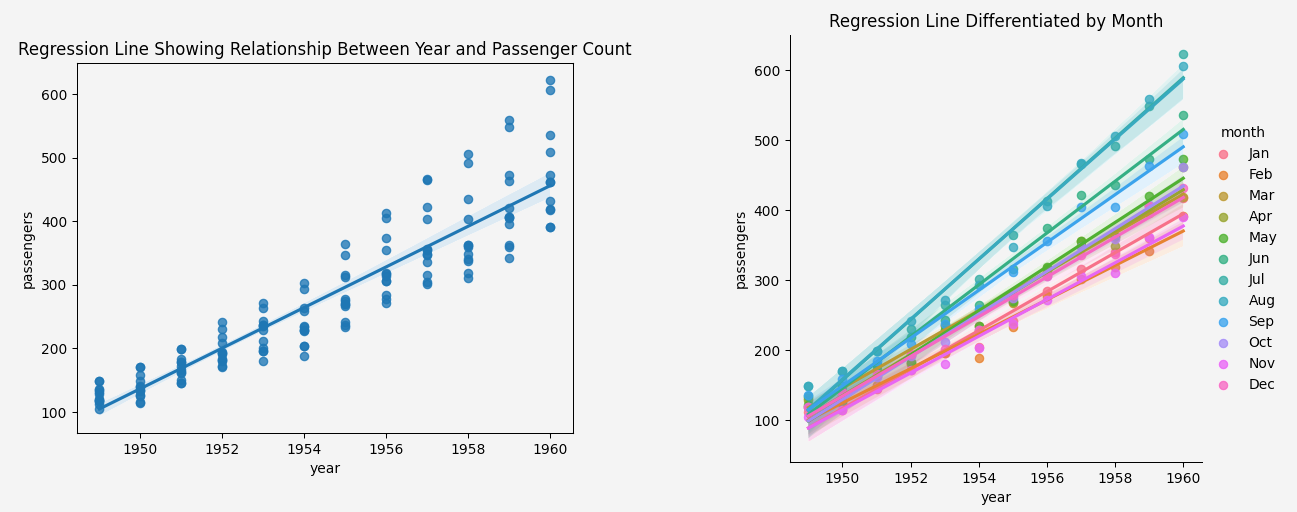Course Content

Data Science Interview Challenge

##Challenge 4: Regression Plots

Exploring the degree and nature of the relationship between variables is foundational in data science. One efficient way to discern this relationship, especially when predicting the behavior of one variable based on another, is through regression plots. Seaborn stands out with its extensive API, equipping users with intuitive tools to visualize regression lines and the spread of data around them.

Regression plots in Seaborn are designed to:

• Analyze the linear relationships between two numeric variables.
• Project potential outcomes based on the regression analysis.
• Highlight the spread or deviation of data points from the regression line.

By harnessing the power of Seaborn's regression plots, practitioners can understand linear relationships, assess the goodness of fit, and make informed predictions.Using Seaborn, showcase the linear relationship in a dataset:

1. Plot a regression line to see how two variables linearly relate.
2. Differentiate data scatter and the regression line based on a categorical variable.
Code Description
`sns.regplot(x=year, y=passengers)`

This code utilizes the `regplot` function from Seaborn to draw a regression line, indicating the linear relationship between the variables `year` and `passengers`. It aids in visualizing the trend and potential predictive capacity between these two variables.

`sns.lmplot(x='year', y='passengers', hue='month', data=data)`

The `lmplot` function is a powerful tool that extends the capability of `regplot`. In this instance, it plots a regression line between `year` and `passengers` but with a twist: the data points and regression lines are differentiated by `months` using the `hue` parameter, allowing for a nuanced understanding of monthly variations.

Everything was clear?

Section 5. Chapter 4